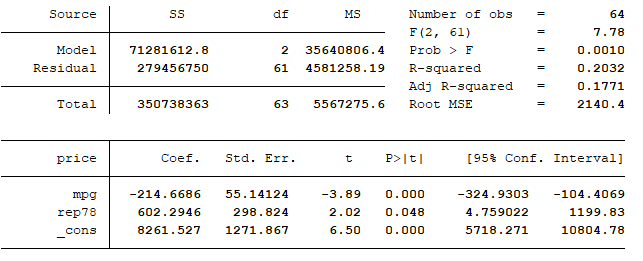Search

# The dfbeta Command - Linear Regression Post-estimation

Updated: Feb 11, 2020

This command generates a dfbeta value for each observation of each independent variable in your regression model. To calculate the dfbeta, Stata compares the coefficient value when an observation is included in the regression model, versus the coefficient value when the same observation is excluded. It does this for the coefficient values of each independent variable in the model. This generates a dfbeta value for each individual observation for each variable.

The dfbeta is used to help identify individual observations that are having an unusually high influence on your model. It is suggested by Belsley, Kuh, and Welsch (1980) that you should look closely at observations where the dfbeta is greater than 2 divided by the square root of N (total number of observations).

## How to Use:

*Perform a regression, then

## Worked Example:

In this example I use the auto dataset. I am going to generate a linear regression, and then use dfbeta to identify individual observations that are skewing my regression model. In the command pane I type the following:

From this I get the following output:The dfbeta command has generated beta values for each observation for mpg (beta1) and rep78 (beta2). The 5 missing values are generated because in the rep78 variable there are 5 observations with a missing rep78 value. Stata excludes observations with missing values when generating a regression model.

Now I want to look specifically for observations that are overly influential. Let’s use the formula from earlier (2/√69). This gives us a value of ~0.241, so we want to look for any beta values that are higher than this. In the command pane I type the following:

This lists the following 8 cars to the results pane:The Cadillac Eldorado and Volvo 260 have a high impact on the coefficient for both mpg and rep78. All three Lincolns, as well as the Mercedes Cougar and the Volkswagen Diesel have a high impact on the coefficient for mpg, but a negligible impact on the rep78 coefficient. Finally the Toyota Corona has a high impact on the rep78 coefficient.

These dfbetas suggest it is alright to leave the Lincoln Continental, Mercedes Cougar and Toyota Corona in the regression model. Though the dfbeta values of these cars are over the 0.241 threshold, they are only just over the cutoff. I am going to exclude the other five cars from the regression model to see if I can get a more uniform model.

The dfbeta values of the cars I think its alright to keep in the model are all under 0.26, and the dfbeta of the cars I want to exclude all easily exceed this value. I use this value as a cutoff to exclude the chosen five cars from my new regression model. In the command pane I type the following:

This gives me the following regression model:Now we compare the two models to see how much of an impact our 5 cars were having on the first model. The best comparison to make is between the R-squared or adjusted-R-squared values for each model. The R-squared value is a measure of how much variance can be explained by your regression model. The adjusted-R-squared value is seen as a more important indicator than the normal R-squared value in multiple linear regressions (linear regressions with more than one independent variable). This is because as you add more and more independent variables to a regression model, the normal R-squared will increase simply because there are more variables. The adjusted-R-squared accounts for this by penalizing you if you include variables with a weak or nonexistent relationship with the dependent variable. As we are looking at a multiple linear regression, we will compare the two adjusted R-squared values.

For the first model the adjusted R-squared is 0.2283, indicating the first model explains about 22.8% of the total variance. For the second model the adjusted R-squared is 0.1771, indicating the second model explains about 17.7% of the total variance. This is a difference of about 5%, with the second model explaining 5% less variance than the first.

This comparison shows the impact of the 5 now-excluded cars is quite significant. The difference between adjusted-R-squared values tells us that 0.0512 of the total 0.2283 from the first model was explained by 5 cars. That difference is about 22% of the total variance the first model explained (0.0512 / 0.2283 * 100).

In the first model there were 69 total observations, of which our 5 cars represented about 7% (5 / 69 * 100). However, that 7% was responsible for explaining about 22% (or just over one fifth) of the total variance the whole model explained. That is a significant influence, and it was giving us an over-positive estimate of the actual amount of variance explained by our regression model.

It is important to use dfbeta to look for overly-influential observations. In this analysis I simply removed any observations I regarded as overly-influential, however this is usually not the best way of dealing with this problem. The observations you identify with this command can include outliers you hadn't yet identified, or other erroneous data. How you deal with these influential observations depends on what you discover about them.